# NCERT Solutions for Chapter 3 How Many Squares? Class 5 Maths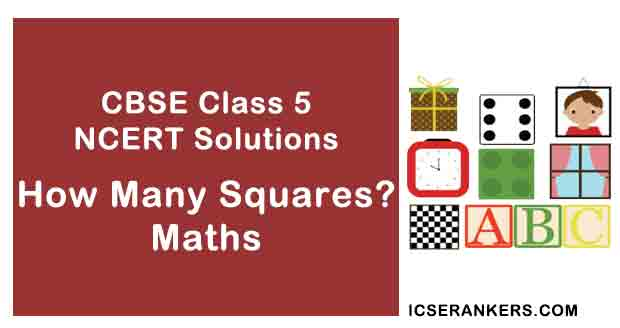How Many Squares? Questions and Answers

 Chapter Name How Many Squares? NCERT Solutions Class CBSE Class 5 Textbook Name Maths Related Readings NCERT Solutions for Class 5NCERT Solutions for Class 5 Maths Summary of How Many Squares?

Page No 34:

Question 1: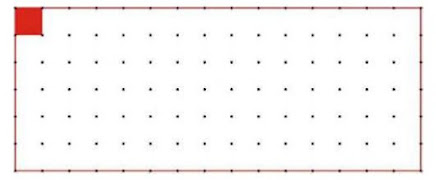• Measure the side of the red square on the dotted sheet. Draw here as many rectangles as possible using 12 such squares.

• How many rectangles could you make?________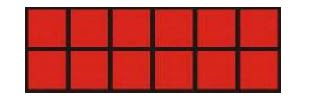Each rectangle is made out of 12 equal squares, so all have the same area, but the length of the boundary will be different.

• Which of these rectangles has the longest perimeter?
• Which of these rectangles has the smallest perimeter?

The length of each side of red square is 1 cm.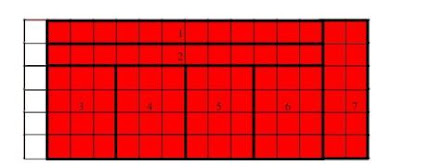• We can make 7 rectangles as shown in the figure.
• The rectangle 1 and rectangle 2 has the longest perimeter.
• The rectangle 3, rectangle 4, rectangle 5 and rectangle 6 has the smallest perimeter.

Page No 35:

Question 1: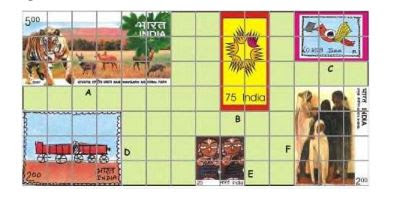Look at these interesting stamps.

(a) How many squares of one centimetre side does stamp A cover? ________ And stamp B? ________

(b) Which stamp has the biggest area? How many squares of side 1 cm does this stamp cover? How much is the area of the biggest stamp? ________  square cm.

(c) Which two stamps have the same area? ________  How much is the area of each of these stamps? ________ square cm.

(d) The area of the smallest stamp is ________ square cm. The

difference between the area of the smallest and the biggest stamp is ________ square cm.

(a) Stamp A covers 18 squares, each of one centimetre side. Stamp B covers 8 squares each of 1 centimetre side.

(b) Stamp A has the biggest area. Stamp A covers 18 squares, each of one centimetre side. Area of biggest stamp = 18 square cm

(c) Stamp D and stamp F has same area. Stamp D and stamp F covers equal number of squares. Each of them covers 12 squares of one centimetre side. Area of each of these stamps 12 square cm

(d) Stamp E is the smallest stamp. It covers 4 squares, each of one centimetre side. Area of smallest stamp = 4 square cm Now, area of biggest stamp = 18 square cm Difference between the area of smallest stamp and biggest stamp = (18-4) square cm = 14 square cm

Page No 36:

Question 1:

(a) Which has the bigger area - one of your footprints or the pages of this book?

(b) Which has the smaller area two five-rupee notes together or a hundred-rupee note?

(c) Look at a 10 rupee-note. Is its area more than hundred square cm?

(d) Is the area of the blue shape more than the area of the yellow shape? Why?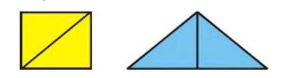(e) Is the perimeter of the yellow shape more than the perimeter of the blue shape? Why?

(a) The pages of this book has a larger area than the footprint.

(b) A hundred-rupee note has smaller area than two five-rupees notes kept together.

(c) The area of a 10-rupee note is less than 100 square cm.

(d) The yellow and blue shaped figures are divided into 2 triangles of equal areas. We now see that the area of each triangle of the blue shape is more than the area of each triangle of the yellow shape. We can then say, that the area of the blue shape is more than the area of the yellow shape.

(e) With the help of a ruler, we come to find that the length of the boundary of the blue shape is more than the length of the boundary of the yellow shape. Thus, we can say that the perimeter of the blue shape is greater than that of the yellow shape.

Question 2: Trace your hand on the squared sheet on the next page. How will you decide whose hand is bigger your hand or your friend's hand?  What is the area of your hand? ________ square cm. What is the area of your friend's hand? ________ square cm

We will trace the hand on the paper and find the area of the paper that gets covered by hand with the help of a square of side 1 cm.

Page No 40:

Question 1: Write the area (in square cm) of the shapes below.We will ignore a square if it is less than half-filled but we will count the square as 1, if it is more than half-filled.

Figure A covers three squares that are more than half-filled, three squares that are less than half-filled, and three complete squares.

We know that an area of one complete square = 1 square cm Area of figure A = area of three more than half-filled squares + area of three complete squares = (3 + 3) square cm = 6 square cm

Figure B covers four complete squares, eight squares that are half-filled, and four squares that are less than half-filled. Area of figure B = area of four complete squares+ area of eight half-filled squares = (4+ 4) square cm = 8 square cm

Figure C covers two complete squares, two squares that are more than half-filled, and two squares that are less than half-filled. Area of figure C = area of two complete squares + area of two squares more than half-filled =(2+2) square cm = 4 square cm

Figure D covers five complete squares and two squares that are half-filled. Area of figure D = area of five complete squares + area of two half-filled squares = (5+1) square cm = 6 square cm

Figure E covers 18 complete squares and six squares that are half-filled. Area of figure E = area of 18 complete squares + area of six half-filled squares = (18+3) square cm = 21 square cm

Figure F covers four squares that are more than half-filled, four squares that are less than half-filled, and four complete squares. Area of figure F = area of four squares more than half-filled squares + area of four complete squares = (4+4) square cm = 8 square cm

Page No 41:

Question 1: The blue triangle is half of the big rectangle. Area of the big rectangle is 20 square cm. So the area of the blue triangle is ­________ square cm.

Area of big rectangle = 20 square cm  Now, it is given that area of blue triangle is half the area of a big rectangle. Area of blue triangle = (20÷2) square cm = 10 square cm

Question 2: Now you find the area of the two rectangles Sadiq is talking about. What is the area of the red triangle? Explain.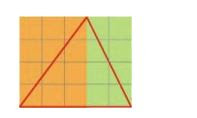There are two rectangles. The orange rectangle contains 12 complete squares and the green rectangle contains 8 complete squares.

Thus, area of an orange rectangle = 12 square cm

Area of green rectangle = 8 square cm Area of the orange portion of a triangle = (12÷2) square cm = 6 square cm

Area of green portion of the triangle = (8÷2) square cm = 4 square cm Area of red triangle = (6+4) square cm = 10 square cm

So, the area of red triangle is 10 square cm.

Page No 42:

Question 1: Suruchi drew two sides of a shape. She asked Asif to complete the shape with two more sides, so that its area is 10 square cm. He completed the shape like this.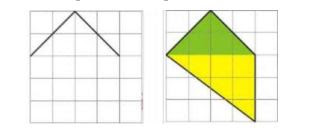• Is the correct? Discuss.
• Explain how the green area is 4 square cm and the yellow area is 6 square cm.

• Yes, Asif is correct.
• We will ignore a square that is less than half-filled, and we will count the square as 1, if it is more than half-filled.

In the green portion, we have two complete squares and four half squares.

Area of one complete square = 1 square cm So, total area of green portion = area of two complete squares + area of four half squares = (2+2) square cm = 4 square cm

In the yellow portion, we have three complete squares, three squares that are more than half-filled, and two squares that are less than half-filled.

Now, area of I complete square = 1 square cm

Area of the yellow portion = area of three complete squares + area of three squares more than half-filled = (3+3) square cm = 6 square cm

Page No 43:

Question 1: Practice time This is one of the sides of a shape. Complete the shape so that its area is 4 square cm.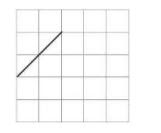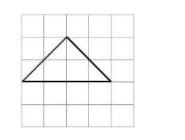Question 2: Practice time Two sides of a shape are drawn here. Complete the shape by drawing two more sides so that its area is less than 2 square cm.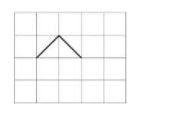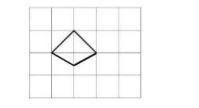The given figure comprises of 2 half-filled squares and 2 squares that are less than half-filled. Thus, we will ignore the squares that are less than half filled.

So, area of the given figure = 1 square cm

Therefore, it clearly proves that the area of the figure i.e. 1 square cm is less than 2 square cm.

Page No 44:

Question 1: Here is a rectangle of area 20 square cm.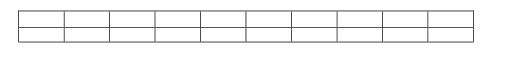(a) Draw one straight line in this rectangle to divide it into two equal triangles. What is the area of each of the triangles?

(b) Draw one straight line in this rectangle to divide it into two equal rectangles. What is the area of each of the smaller rectangles?

(c) Draw two straight lines in this rectangle to divide it into one rectangle and two equal triangles.

• What is the area of the rectangle?
• What is the area of each of the triangles?

(a) The given rectangle is divided into two equal triangles by drawing a line as shown below.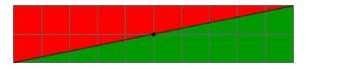Area of rectangle = 20 square cm Area of each triangle =

12of area of rectangle = (20÷2) square cm = 10 square cm

(b) The given rectangle is divided into two equal rectangles by drawing a line as shown below.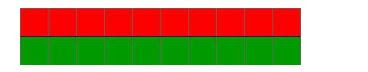Area of rectangle = 20 square cm Thus, area of each small rectangle =

12of area of rectangle = (20÷ 2) square cm = 10 square cm

(c) The given rectangle is divided into one rectangle and two equal triangles by drawing 2 lines as shown below.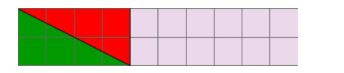We will consider a square as complete if it is more than half-filled, and will ignore a square if it is less than half-filled.

Thus, in the red shaded region, we have 2 completely filled squares and 2 squares that are more than half-filled. Thus, the area of red triangle = 4 square cm

Similarly, the area of green triangle = 4 square cm Now, the area of remaining portion i.e. the rectangle contains 12 completely filled squares.

• Thus, the area of rectangle = 12 square cm. Area of each of the triangle is 4 square cm.

Disclaimer: The answer to part (c) of the question may vary from student to student. The answer provided here is for reference only.

Page No 45:

Question 1: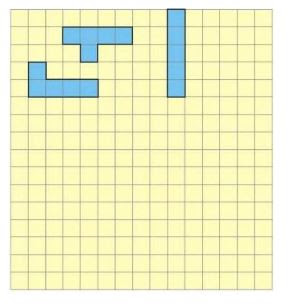(a) How many different shapes can you draw? ________

(b) Which shape has the longest perimeter? How much? ________ cm

(c) Which shape has the shortest perimeter? How much? ________cm

(d) What is the area of the shapes? ________ square cm. That's simple!

(a) There are 12 different shapes that can be drawn using 5 squares.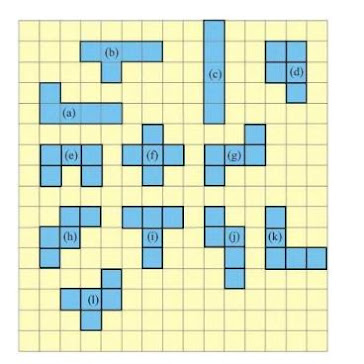(b) Among all the 12 shapes, there is only one shape, figure (d) that has the smallest perimeter, but all the other shapes have same and longest perimeter.

Longest perimeter of each 11 shapes = (1 + 1 + 1 +1 + 1 + 1 + 1 + 1 + 1 + 1 + 1 + 1) cm = 12 cm

(c) The figure (d) has the smallest perimeter. Perimeter of figure (d) = (1 + 1 + 1 + 1 + 1 + 1 + 1 + 1 + 1 + 1) cm = 10 cm

(d) We find that each of the 12 shapes covers 5 complete squares. Area of 1 complete square = 1 square cm Area of 5 complete squares = 1 × 5 square cm = 5 square cm

Area of each shape = Area of 5 complete squares = 5 square cm

Page No 49:

Question 1: Practice time Ziri tried to make some tiles. She started with a square of 2 cm side and made shapes like these.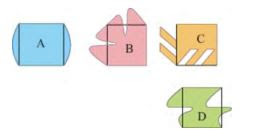Look at these carefully and find out: •  Which of these shapes will tile a floor (without any gaps)? Discuss. What is the area of these shapes? • Make designs in your copy by tiling those shapes.

The shapes C and D will tile a floor without leaving any gaps. Area of each shape = 2 × 2 = 4 square cm

Design with shape C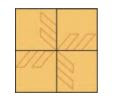Design with shape D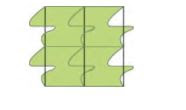Disclaimer: The answer may vary from student to student. It is highly

recommended that the students prepare the answer on their own.